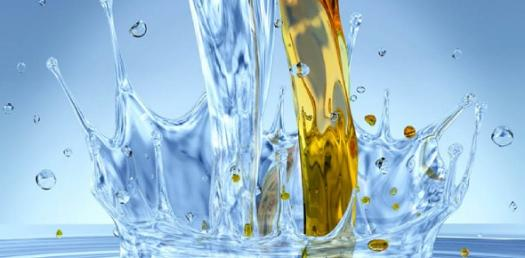# Quiz Questions Over The Properties Of Water

10 Questions | Total Attempts: 2589SettingsThis quiz checks students basic knowledge of the properties of water.

• 1.
Which atom/s of the water molecule has a slightly positive charge?
• A.

Oxygen

• B.

Helium

• C.

Hydrogen

• D.

Lithium

• E.

Ozone

• 2.
Which atom/s of a water molecule has a slightly negative charge?
• A.

Oxygen

• B.

Helium

• C.

Hydrogen

• D.

Lithium

• E.

Ozone

• 3.
A molecule that has electrically charged areas is called a ____________________ ?
• A.

Solution

• B.

Solvent

• C.

Specific Heat

• D.

Polar Molecule

• E.

Charged Particle

• 4.
The opposite poles of water molecules are attracted to each other and causes water molecules to "stick" together.  This force is _________________ ?
• A.

Potential

• B.

• C.

Nuclear

• D.

Magnetic

• E.

Electrical

• 5.
Which property of water that causes it to rise in a tube?
• A.

Surface tension

• B.

High specific heat

• C.

Universal solvent

• D.

Capillary action

• E.

Changing state

• 6.
Which property of water allows the insect called a water stryder to skate across the surface of a pond?
• A.

Surface tension

• B.

High specific heat

• C.

Universal solvent

• D.

Capillary action

• E.

Changing state

• 7.
When one substance dissolves in another it is a ________________ ?
• A.

Solvent

• B.

Capillary action

• C.

Specific heat

• D.

Solution

• E.

Surface tension

• 8.
The temperature in Celsius in which water freezes?
• A.

212

• B.

100

• C.

32

• D.

0

• E.

50

• 9.
The temperature in Celsius in which water boils?
• A.

212

• B.

100

• C.

32

• D.

0

• E.

50

• 10.
The process in which liquid water becomes a gas is called evaporation.
• A.

True

• B.

False

Related TopicsBack to top